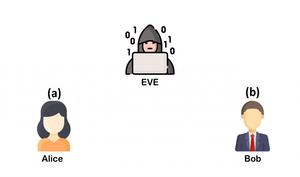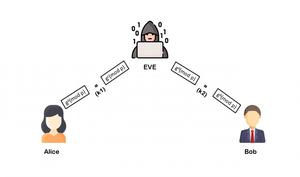Related Articles

# Man in the Middle attack in Diffie-Hellman Key Exchange

• Last Updated : 18 Jul, 2021

Prerequisite: Diffie-Hellman Algorithm

Diffie-Hellman Key Exchange algorithm is an advanced cryptographic method used to establish a shared secret (or shared secret key) that can be used to perform secret communication on a public network between Alice and Bob while preventing Eve (eavesdropper), who can eavesdrop on all their communication, from learning the generated secret.

The key exchange procedure has two steps :

1. One-time setup: We define some public parameters that are used by everyone forever.
2. Protocol: To generate new secret keys, run a two-message key exchange protocol. This process is done using some simple algebra, prime numbers, and properties of modular arithmetic.

## Security Threat of the Diffie-Hellman

Let’s assume that the eavesdropper EVE knows the public values p and g like everyone else, and from her eavesdropping, she learns the values exchanged by Alice and Bob, gᵃ mod p and gᵇ mod p, as well. With all her knowledge, she still can’t compute the secret key S, as it turns out, if p and g are properly chosen, it’s very, very hard for her to do.

For instance, you could brute force it and try all the options, but The calculations (mod p) make the discrete log calculation super slow when the numbers are large. If p and g have thousands of bits, then the best-known algorithms to compute discrete logs, although faster than plain brute force, will still take millions of years to compute.

Even with its immunity to brute force, it’s vulnerable to MITM (man in the middle position).

### Man in the Middle (MITM) against Diffie-Hellman:

A malicious Malory, that has a MitM (man in the middle) position, can manipulate the communications between Alice and Bob, and break the security of the key exchange.

Step by Step explanation of this process:

Step 1: Selected public numbers p and g, p is a prime number, called the “modulus” and g is called the base.

Step 2: Selecting private numbers.

let Alice pick a private random number a and let Bob pick a private random number b, Malory picks 2 random numbers c and d.Step 3: Intercepting public values,

Malory intercepts Alice’s public value (ga(mod p)), block it from reaching Bob, and instead sends Bob her own public value (gc(modp)) and Malory intercepts Bob’s public value (gb(mod p)), block it from reaching Alice, and instead sends Alice her own public value (gd (modp))Step 4: Computing secret key

Alice will compute a key S1=gda(mod p), and Bob will compute a different key, S2=gcb(mod p)Step 5: If Alice uses S1 as a key to encrypt a later message to Bob, Malory can decrypt it, re-encrypt it using S2, and send it to Bob. Bob and Alice won’t notice any problem and may assume their communication is encrypted, but in reality, Malory can decrypt, read, modify, and then re-encrypt all their conversations.

Below is the implementation:

## Python3

 `import` `random`` ` `# public keys are taken``# p is a prime number``# g is a primitive root of p``p ``=` `int``(``input``(``'Enter a prime number : '``))``g ``=` `int``(``input``(``'Enter a number : '``))`` ` ` ` `class` `A:``    ``def` `__init__(``self``):``        ``# Generating a random private number selected by alice``        ``self``.n ``=` `random.randint(``1``, p)     `` ` `    ``def` `publish(``self``):``        ``# generating public values``        ``return` `(g``*``*``self``.n)``%``p`` ` `    ``def` `compute_secret(``self``, gb):``        ``# computing secret key``        ``return` `(gb``*``*``self``.n)``%``p`` ` ` ` `class` `B:``    ``def` `__init__(``self``):``        ``# Generating a random private number selected for alice``        ``self``.a ``=` `random.randint(``1``, p)``        ``# Generating a random private number selected for bob``        ``self``.b ``=` `random.randint(``1``, p)``        ``self``.arr ``=` `[``self``.a,``self``.b]`` ` `    ``def` `publish(``self``, i):``        ``# generating public values``        ``return` `(g``*``*``self``.arr[i])``%``p`` ` `    ``def` `compute_secret(``self``, ga, i):``        ``# computing secret key``        ``return` `(ga``*``*``self``.arr[i])``%``p`` ` ` ` `alice ``=` `A()``bob ``=` `A()``eve ``=` `B()`` ` `# Printing out the private selected number by Alice and Bob``print``(f``'Alice selected (a) : {alice.n}'``)``print``(f``'Bob selected (b) : {bob.n}'``)``print``(f``'Eve selectd private number for Alice (c) : {eve.a}'``)``print``(f``'Eve selectd private number for Bob (d) : {eve.b}'``)`` ` `# Generating public values ``ga ``=` `alice.publish()``gb ``=` `bob.publish()``gea ``=` `eve.publish(``0``)``geb ``=` `eve.publish(``1``)``print``(f``'Alice published (ga): {ga}'``)``print``(f``'Bob published (gb): {gb}'``)``print``(f``'Eve published value for Alice (gc): {gea}'``)``print``(f``'Eve published value for Bob (gd): {geb}'``)`` ` `# Computing the secret key``sa ``=` `alice.compute_secret(gea)``sea ``=` `eve.compute_secret(ga,``0``)``sb ``=` `bob.compute_secret(geb)``seb ``=` `eve.compute_secret(gb,``1``)``print``(f``'Alice computed (S1) : {sa}'``)``print``(f``'Eve computed key for Alice (S1) : {sea}'``)``print``(f``'Bob computed (S2) : {sb}'``)``print``(f``'Eve computed key for Bob (S2) : {seb}'``)`

Output:

```Enter a prime number (p) : 227
Enter a number (g) : 14

Alice selected (a) : 227
Bob selected (b) : 170

Eve selectd private number for Alice (c) : 65
Eve selectd private number for Bob (d) : 175

Alice published (ga): 14
Bob published (gb): 101

Eve published value for Alice (gc): 41
Eve published value for Bob (gd): 32

Alice computed (S1) : 41
Eve computed key for Alice (S1) : 41

Bob computed (S2) : 167
Eve computed key for Bob (S2) : 167```

Attention geek! Strengthen your foundations with the Python Programming Foundation Course and learn the basics.

To begin with, your interview preparations Enhance your Data Structures concepts with the Python DS Course. And to begin with your Machine Learning Journey, join the Machine Learning – Basic Level Course

My Personal Notes arrow_drop_up